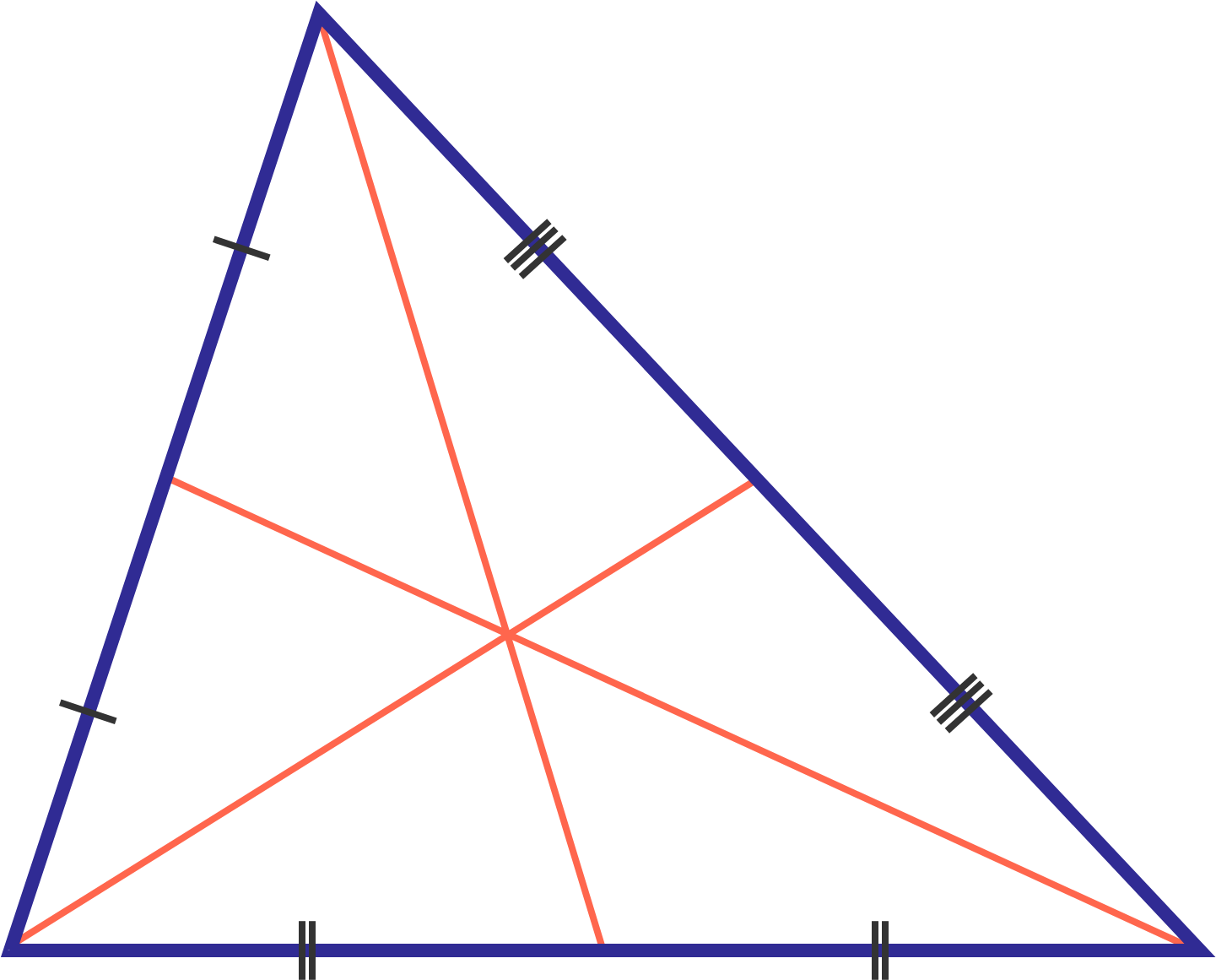# Median Triangles!

Geometry Level 1If the medians of triangle $ABC$ intersect at $O$, then evaluate $\frac{AB^2+BC^2+AC^2}{OA^2+OB^2+OC^2}$.

×